JEE  >  DC Pandey Solutions: Laws of Motion- 3

# DC Pandey Solutions: Laws of Motion- 3 - Notes | Study DC Pandey Solutions for JEE Physics - JEE

 1 Crore+ students have signed up on EduRev. Have you?

Introductory Exercise 5.5

Ques 1: In figure m1 = 1 kg and m2 = 4 kg. Find the mass M o f the hanging block which will prevent the smaller block from slipping over the triangular block. All the surfaces are friction less and the strings and the pulleys are light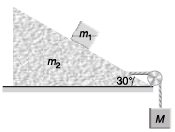Note In exercises 2 to 4 the situations described take place in a box car which has initial velocity v = 0 but acceleration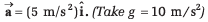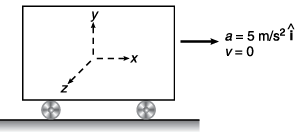Ans: 6 .83 kg
Sol: Block on triangular block will not slip if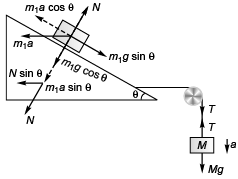m1a cos θ= m1g sin θ
i.e., a = g tanθ …(i)
N = m1g cosθ + m1asinθ …(ii)
For the movement of triangular block
T - N sinθ = m2α …(iii)
For the movement of the block of mass M
Mg - T = Ma …(iv)
Mg - N sinθ = ( m2 + M )α
Substituting the value of N from Eq. (ii) in the above equation
Mg - (m1g cosθ + m1α sinθ) sinθ
=( m2α + Mα)
i.e., M (g - α) = m1g cosθ sinθ + (m2 + m1 sin2θ)α
Substituting value of a from Eq. (i) in the above equation,
M(1 - tanθ) = m1 cosθ sinθ + ( m2 + m1 sin2 θ) tanθ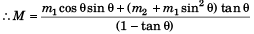Substituting θ = 30° , m1 = 1 kg and m2 = 4 kg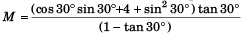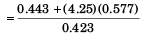= 6.82 kg

Ques 2: A 2 kg object is slid along the friction less floor with initial velocity (10 m/s)î (a) Describe the motion of the object relative to car (b) when does the object reach its original position relative to the box car.
Ans:
(a) x = x0 + 10t - 2.5t2 , v = 10 - 5t (b) t = 4s
Sol: (a) Using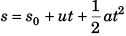Displacement of block at time t relative to car would be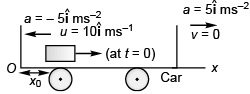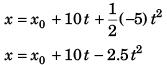Velocity of block at time t (relative to car) will be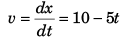(b) Time (t) for the block to arrive at the original position (i.e., x = x0) relative to car
x0 = x+ 10 t - 2.5 t2
⇒ t = 4s

Ques 3: A 2 kg object is slid along the friction less floor with initial transverse velocity (10 m/s)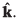. Describe the motion (a) in car’s frame (b) in ground frame.
Ans:
(a) x = x0 - 2.5t2, z = z0 + 10t, vx = - 5t, vz = 10 ms-1
(b) x = x0, z = z0 + 10t, vx = 0, vz = 10 ms-1
Sol: (a) In car’s frame position of object at time t would be given by
In car’s frame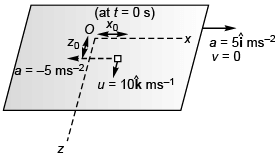x = x0 + 0*t + 1/2(-5)t2
i.e., x = x0 - 2.5 t2 …(i)
and z = z + 10t …(ii)
Velocity of the object at time t would be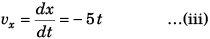and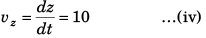(b) In ground frame the position of the object at time t would be given by
In ground frame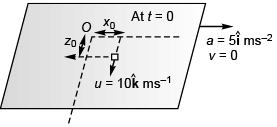x = x0
and z = z0 + 10t
Velocity of the object at time t would be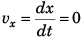ans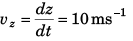Ques 4: A 2 kg object is slid along a rough floor (coefficient of sliding friction = 0.3) with initial velocity (10 m/s)î. Describe the motion of the object relative to car assuming that the coefficient of static friction is greater than 0.5.
Ans:
x = x0 + 10f - 4t2 , K = 10 - 8 t for 0<f<1.25 s object stops at t = 1.25 s and remains at rest relative to car.
Sol: m = 2 kg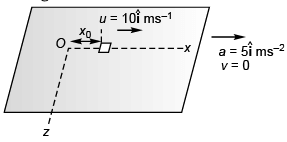Normal force on object = mg
Maximum sliding friction = μsmg
= 0.3*2*10 = 6 N
Deceleration due to friction = 6/2 =3 m/s2
Deceleration due to pseudo force  = 5 m/s2
∴ Net deceleration = (3 + 5) m/s2
= 8 m/s2
∴ Displacement of object at any time t (relative to car)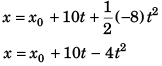Thus, velocity of object at any time t (relative to car)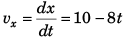The object will stop moving relative to car when
10 - 8t = 0 i.e., t = 1.25s
∴ vx = 10 - 8t for  0<t<1.25 s

Ques 5: A block is placed on an inclined plane as shown in figure. What must be the frictional force between block and incline if the block is not to slide along the incline when the incline is accelerating to the right at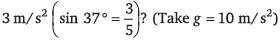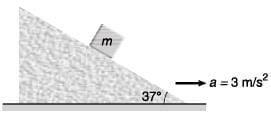Ans:
9/25 mg
Sol: For block not to slide the frictional force ( f ) would be given by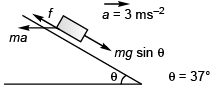f + ma cosθ = mg sinθ
or
f = mg sinθ - ma cosθ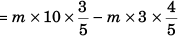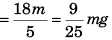The document DC Pandey Solutions: Laws of Motion- 3 - Notes | Study DC Pandey Solutions for JEE Physics - JEE is a part of the JEE Course DC Pandey Solutions for JEE Physics.
All you need of JEE at this link: JEE

210 docs

## DC Pandey Solutions for JEE Physics

210 docs

Track your progress, build streaks, highlight & save important lessons and more!

,

,

,

,

,

,

,

,

,

,

,

,

,

,

,

,

,

,

,

,

,

;